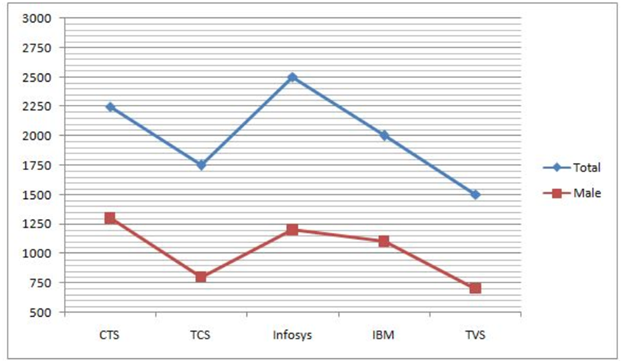# RRB Clerk Prelims Quantitative Aptitude Questions 2021 (Day-08)

Dear Aspirants, Our IBPS Guide team is providing new series of Quantitative Aptitude Questions for RRB Clerk Prelims 2021 so the aspirants can practice it on a daily basis. These questions are framed by our skilled experts after understanding your needs thoroughly. Aspirants can practice these new series questions daily to familiarize with the exact exam pattern and make your preparation effective.

Start Quiz

Simplification

Directions (01-05): What value should come in the place of (?) in the following questions?

1) 50% of (384 – 720/2) * 40 = ?

A.825

B.480

C.925

D.638

E.352

2) (0.512)(2/5) * (0.8)3 ÷ (0.4096) = (0.8)(?/5)

A.1

B.2

C.3

D.0

E.4

3) 30 % of 360 + 75% of 80 + 83.33 % of 240 = ?

A.388

B.368

C.378

D.358

E.348

4) 49 * 3 + 70% of 60 = ? + 102

A.89

B.150

C.172

D.250

E.314

5) (80% of 6450) ÷ 40 + 36 =?

A.90

B.100

C.165

D.189

E.210

Line graph

Directions (06-10): Study the following information carefully and answer the questions given below.

The given line graph shows the total number of employees and the number of male employees in five different companies.6) What is the average number of female employees in all the companies together?

A.920

B.950

C.970

D.980

E.940

7) What is the ratio of the total number of employees in Infosys and TVS together to the total number of male employees in CTS and IBM together?

A.2:1

B.3:2

C.5:3

D.6:5

E.3:1

8) The number of male employees in Apple is 60% more than the number of male employees in TVS and the number of male to female employees in Apple in the ratio of 8:7. What is the total number of employees in Apple?

A.2250

B.2200

C.2100

D.2300

E.2350

9) The total number of employees in TCS is what percent more than the number of female employees in CTS?

A.87.4%

B.82.1%

C.85.3%

D.84.2%

E.80.4%

10) What is the average number of employees in all the companies together?

A.1600

B.1700

C.1800

D.1900

E.None of these

=> 50% of (384 – 360) * 40

=> 480

(0.512)(2/5) * (0.8)3 ÷ (0.4096) = (0.8)(?/5)

(0.8)6/5 – 1 = (0.8)(?/5)

1/5 = ?/5

? = 1

30 % of 360 + 75% of 80 + 83.33 % of 240 = ?

108 + 60 + (5/6) * 240 = ?

108 + 60 + 200 = ?

368 = ?

=> 49 * 3 + 70/100 * 60 = ? + 100

=> 189 – 100

=> 89

=> (80% of 6450)/40 + 36 = ?

=> 165

Number of female employees in CTS = 2250 – 1300 = 950

Number of female employees in TCS = 1750 – 800 = 950

Number of female employees in Infosys = 2500 – 1200 = 1300

Number of female employees in IBM = 2000 – 1100 = 900

Number of female employees in TVS = 1500 – 700 = 800

Required Average = (950 + 950 + 1300 + 900 + 800)/5 = 980

Required ratio = (2500 + 1500):(1300+1100)

=4000:2400

=5:3

Male employees in apple = 700 * 160/100 = 1120

Total number of employees in Apple = 15/8 * 1120 = 2100

Required % = (1750 – 950)/950 * 100 = 84.21%